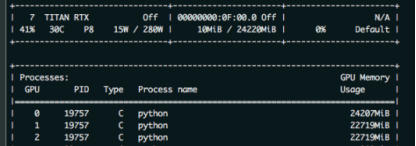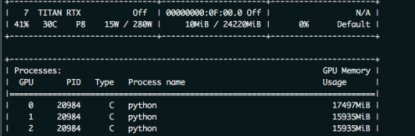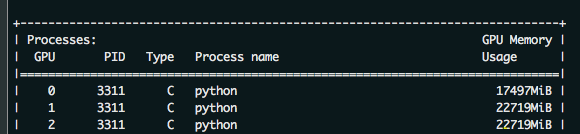# Solution to unbalanced load of multiple cards (GPU’s 0 card is too high) in Python model training (simple and effective)

this paper mainly solves the problem that zero card of pytorch GPU occupies more video memory than other CARDS during model training. As shown in the figure below: the native GPU card is TITAN RTX, video memory is 24220M, batch_size = 9, and three CARDS are used. The 0th card video memory occupies 24207M. At this time, it just starts to run, and only a small amount of data is transferred to the video card. If the data is in multiple points, the video memory of the 0 card must burst. The reason why 0 card has higher video memory: During the back propagation of the network, the calculated gradient of loss is calculated on 0 card by default. So will be more than other graphics card some video memory, how much more specific, mainly to see the structure of the network.as a result, in order to prevent training was interrupted due to out of memory. The foolhardy option is to set batch_size to 6, or 2 pieces of data per card.
batch_size = 6, the other the same, as shown in the figure belowhave found the problem?Video memory USES only 1,2 CARDS and less than 16 gigabytes of memory. The batch_size is sacrificed because the 0 card might exceed a little bit of video memory.
so there’s no more elegant way?The answer is yes. That is borrowed from the transformer – xl BalancedDataParallel used in the class. The code is as follows (source) :

``````import torch
from torch.nn.parallel.data_parallel import DataParallel
from torch.nn.parallel.parallel_apply import parallel_apply
from torch.nn.parallel._functions import Scatter

def scatter(inputs, target_gpus, chunk_sizes, dim=0):
r"""
Slices tensors into approximately equal chunks and
distributes them across given GPUs. Duplicates
references to objects that are not tensors.
"""

def scatter_map(obj):
if isinstance(obj, torch.Tensor):
try:
return Scatter.apply(target_gpus, chunk_sizes, dim, obj)
except Exception:
print('obj', obj.size())
print('dim', dim)
print('chunk_sizes', chunk_sizes)
quit()
if isinstance(obj, tuple) and len(obj) > 0:
return list(zip(*map(scatter_map, obj)))
if isinstance(obj, list) and len(obj) > 0:
return list(map(list, zip(*map(scatter_map, obj))))
if isinstance(obj, dict) and len(obj) > 0:
return list(map(type(obj), zip(*map(scatter_map, obj.items()))))
return [obj for targets in target_gpus]

# After scatter_map is called, a scatter_map cell will exist. This cell
# has a reference to the actual function scatter_map, which has references
# to a closure that has a reference to the scatter_map cell (because the
# fn is recursive). To avoid this reference cycle, we set the function to
# None, clearing the cell
try:
return scatter_map(inputs)
finally:
scatter_map = None

def scatter_kwargs(inputs, kwargs, target_gpus, chunk_sizes, dim=0):
"""Scatter with support for kwargs dictionary"""
inputs = scatter(inputs, target_gpus, chunk_sizes, dim) if inputs else []
kwargs = scatter(kwargs, target_gpus, chunk_sizes, dim) if kwargs else []
if len(inputs) < len(kwargs):
inputs.extend([() for _ in range(len(kwargs) - len(inputs))])
elif len(kwargs) < len(inputs):
kwargs.extend([{} for _ in range(len(inputs) - len(kwargs))])
inputs = tuple(inputs)
kwargs = tuple(kwargs)
return inputs, kwargs

class BalancedDataParallel(DataParallel):

def __init__(self, gpu0_bsz, *args, **kwargs):
self.gpu0_bsz = gpu0_bsz
super().__init__(*args, **kwargs)

def forward(self, *inputs, **kwargs):
if not self.device_ids:
return self.module(*inputs, **kwargs)
if self.gpu0_bsz == 0:
device_ids = self.device_ids[1:]
else:
device_ids = self.device_ids
inputs, kwargs = self.scatter(inputs, kwargs, device_ids)
if len(self.device_ids) == 1:
return self.module(*inputs, **kwargs)
replicas = self.replicate(self.module, self.device_ids)
if self.gpu0_bsz == 0:
replicas = replicas[1:]
outputs = self.parallel_apply(replicas, device_ids, inputs, kwargs)
return self.gather(outputs, self.output_device)

def parallel_apply(self, replicas, device_ids, inputs, kwargs):
return parallel_apply(replicas, inputs, kwargs, device_ids)

def scatter(self, inputs, kwargs, device_ids):
bsz = inputs.size(self.dim)
num_dev = len(self.device_ids)
gpu0_bsz = self.gpu0_bsz
bsz_unit = (bsz - gpu0_bsz) // (num_dev - 1)
if gpu0_bsz < bsz_unit:
chunk_sizes = [gpu0_bsz] + [bsz_unit] * (num_dev - 1)
delta = bsz - sum(chunk_sizes)
for i in range(delta):
chunk_sizes[i + 1] += 1
if gpu0_bsz == 0:
chunk_sizes = chunk_sizes[1:]
else:
return super().scatter(inputs, kwargs, device_ids)
return scatter_kwargs(inputs, kwargs, device_ids, chunk_sizes, dim=self.dim)
``````

you can see, in the code BalancedDataParallel inherited the torch. The nn. DataParallel, through the custom after 0, the size of the card batch_size gpu0_bsz, namely 0 card a bit less data. Balance the memory usage of 0 CARDS with other CARDS. The invocation code is as follows:

``````import BalancedDataParallel

if n_gpu > 1:
model = BalancedDataParallel(gpu0_bsz=2, model, dim=0).to(device)
# model = torch.nn.DataParallel(model)
``````

gpu0_bsz: 0 card batch_size of GPU;
model: model;
dim: batch dimension

as a result, we might as well just batch_size set to 8, namely gpu0_bsz = 2 try, the results are as follows:the batch_size from 6 to 8 of success, because 0 put a batch less, therefore, will be smaller than the other CARDS. But sacrificing the video memory of one card to the video memory of others, eventually increasing the batch_size, is still available. The advantages of this method are even more obvious when the number of CARDS is large.